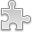# Courses / Module

Toggle Print

##CODING THEORY

Module code: MT314S
Credits: 5
Semester: 1
Department: MATHEMATICS AND STATISTICS
International:Overview

Module Objective:
To introduce students to Coding Theory.

Selected Topics from the following:
Introduction of information transmission and error detection. Binary encoding of messages. Hamming distance. Error-correcting codes over finite alphabets. Sphere-packing bound, perfect codes. Gibert-Varshamov bound. The coding theory problem. Error probabilities. Discussion of Shannon�s theorem.
Linear codes. Equivalence of codes. Generator matrix, row operations modulo p and the standard form, encoding. Parity check matrix. Hamming codes. Syndrome decoding. Dual code.
Cyclic codes and polynomials modulo xn-1. Generator polynomial. Linear recursions and cyclic codes. Weight enumerator, MacWilliams identities. Reed-Muller codes. Golay codes. Reed-Solomon Codes and Compact Disc player. Convolutional codes.
Computation using mathematical/statistical software.Learning OutcomesTeaching & Learning methodsAssessmentAutumn Supplementals/ResitsPre-RequisitesTimetable